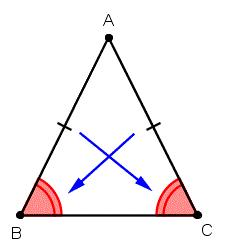# Circumference 4430

In an isosceles triangle with a circumference of 36 cm, the height at the base is 12 cm long. Calculate the length of the arm of a given triangle.

r =  13 cm

### Step-by-step explanation:Did you find an error or inaccuracy? Feel free to write us. Thank you!

Tips for related online calculators
Are you looking for help with calculating roots of a quadratic equation?
The Pythagorean theorem is the base for the right triangle calculator.